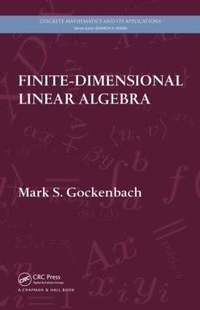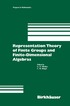Fler böcker inom
Format
Inbunden (Hardback)
Språk
Engelska
Antal sidor
672
Utgivningsdatum
2010-05-10
Förlag
CRC Press Inc
Illustratör/Fotograf
8 black & white tables 50 black & white illustrations
Illustrationer
N/A; 8 Tables, black and white; 50 Illustrations, black and white
Dimensioner
236 x 157 x 36 mm
Vikt
1112 g
Antal komponenter
1
Komponenter
52:B&W 6.14 x 9.21in or 234 x 156mm (Royal 8vo) Case Laminate on White w/Gloss Lam
ISBN
9781439815632

### Du kanske gillar# Finite-Dimensional Linear Algebra

Inbunden Engelska, 2010-05-10
979
Skickas inom 5-8 vardagar.
Gratis frakt inom Sverige över 159 kr för privatpersoner.
Finns även som
879
Linear algebra forms the basis for much of modern mathematics-theoretical, applied, and computational. Finite-Dimensional Linear Algebra provides a solid foundation for the study of advanced mathematics and discusses applications of linear algebra to such diverse areas as combinatorics, differential equations, optimization, and approximation. The author begins with an overview of the essential themes of the book: linear equations, best approximation, and diagonalization. He then takes students through an axiomatic development of vector spaces, linear operators, eigenvalues, norms, and inner products. In addition to discussing the special properties of symmetric matrices, he covers the Jordan canonical form, an important theoretical tool, and the singular value decomposition, a powerful tool for computation. The final chapters present introductions to numerical linear algebra and analysis in vector spaces, including a brief introduction to functional analysis (infinite-dimensional linear algebra). Drawing on material from the author's own course, this textbook gives students a strong theoretical understanding of linear algebra. It offers many illustrations of how linear algebra is used throughout mathematics.

## Passar bra ihop

1.2. +
3.De som köpt den här boken har ofta också köpt Representation Theory of Finite Groups and Fini... av Michler, Ringel (inbunden).

## Kundrecensioner

Har du läst boken? Sätt ditt betyg »

## Fler böcker av Mark S Gockenbach

• ### Understanding and Implementing the Finite Element Method

#### Mark S Gockenbach

The finite element method is the most powerful general-purpose technique for computing accurate solutions to partial differential equations. This book contains a thorough derivation of the finite element equations as well as sections on programmin...

• ### Partial Differential Equations

#### Mark S Gockenbach

Undergraduate courses on partial differential equations (PDEs) have traditionally been based on the Fourier series method for analysing and solving PDEs. What this textbook offers is a fresh approach; the traditional method taught alongside the mo...

## Övrig information

Mark S. Gockenbach is a professor and chair of the Department of Mathematical Sciences at Michigan Technological University.

## Innehållsförteckning

Some Problems Posed on Vector Spaces Linear equations Best approximation Diagonalization Summary Fields and Vector Spaces Fields Vector spaces Subspaces Linear combinations and spanning sets Linear independence Basis and dimension Properties of bases Polynomial interpolation and the Lagrange basis Continuous piecewise polynomial functions Linear Operators Linear operators More properties of linear operators Isomorphic vector spaces Linear operator equations Existence and uniqueness of solutions The fundamental theorem; inverse operators Gaussian elimination Newton's method Linear ordinary differential equations (ODEs) Graph theory Coding theory Linear programming Determinants and Eigenvalues The determinant function Further properties of the determinant function Practical computation of det(A) A note about polynomials Eigenvalues and the characteristic polynomial Diagonalization Eigenvalues of linear operators Systems of linear ODEs Integer programming The Jordan Canonical Form Invariant subspaces Generalized eigenspaces Nilpotent operators The Jordan canonical form of a matrix The matrix exponential Graphs and eigenvalues Orthogonality and Best Approximation Norms and inner products The adjoint of a linear operator Orthogonal vectors and bases The projection theorem The Gram-Schmidt process Orthogonal complements Complex inner product spaces More on polynomial approximation The energy inner product and Galerkin's method Gaussian quadrature The Helmholtz decomposition The Spectral Theory of Symmetric Matrices The spectral theorem for symmetric matrices The spectral theorem for normal matrices Optimization and the Hessian matrix Lagrange multipliers Spectral methods for differential equations The Singular Value Decomposition Introduction to the singular value decomposition (SVD) The SVD for general matrices Solving least-squares problems using the SVD The SVD and linear inverse problems The Smith normal form of a matrix Matrix Factorizations and Numerical Linear Algebra The LU factorization Partial pivoting The Cholesky factorization Matrix norms The sensitivity of linear systems to errors Numerical stability The sensitivity of the least-squares problem The QR factorization Eigenvalues and simultaneous iteration The QR algorithm Analysis in Vector Spaces Analysis in Rn Infinite-dimensional vector spaces Functional analysis Weak convergence Appendix A: The Euclidean Algorithm Appendix B: Permutations Appendix C: Polynomials Appendix D: Summary of Analysis in R Bibliography Index